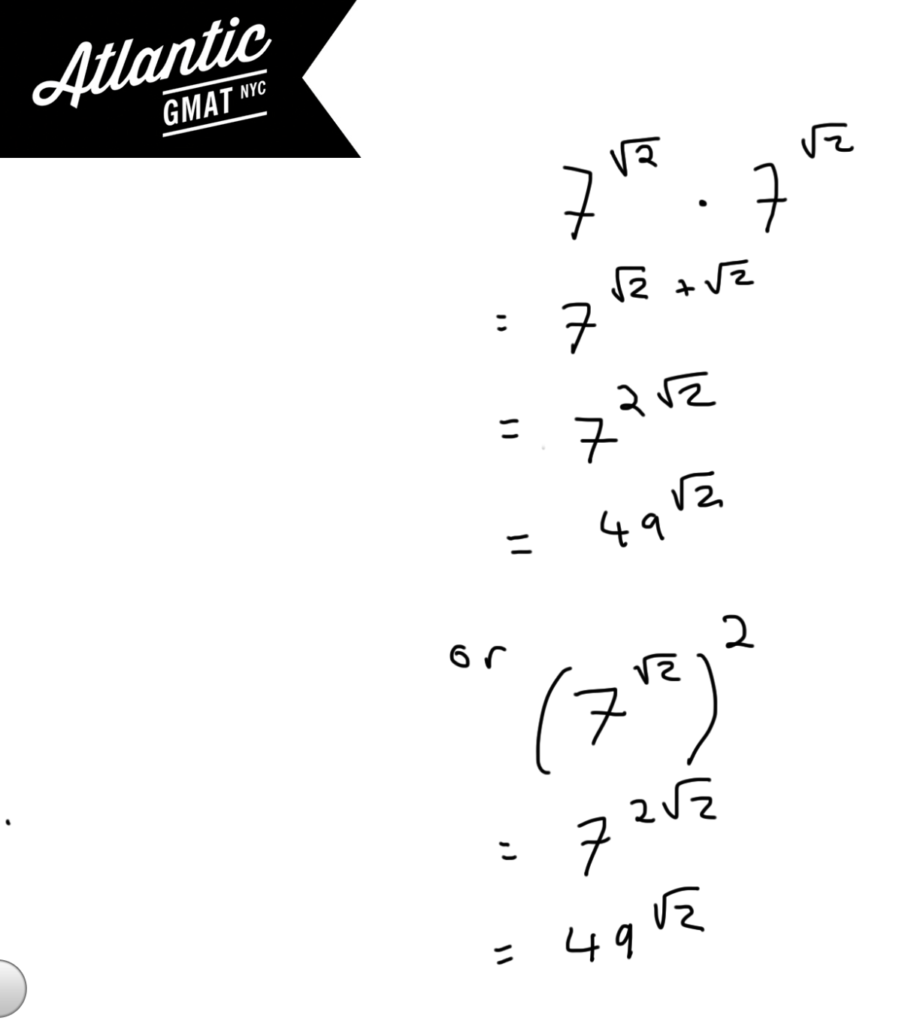GMAT Question of the Day - Problem Solving - Exponents

What is the square of 7^√2?

A. 7

B. 14

C. 14√2

D. 49

E. 49√2

GMAT Question of the Day Solution

GMAT exponents questions only require that you follow a simple set of rules. The difficulty in these questions is that the GMAT will present strange numbers which might tempt you to stray from the rules. Almost every day while I am GMAT tutoring I encourage students to follow the basic rules regardless of what oddball question the GMAT throws at them. It can take some time to trust the fact that the simple rules will unravel even the most complex expressions but trust me - they will. In this question of the day we need to raise the expression by a power of two. You should be thinking: hmmm raising to the power of two that means that I multiply the exponent by two. Bingo. Now we just have to make sure that we have our answer in the same format as the actual answer.Sorry, comments are closed for this post.

CONTACT

Atlantic GMAT

405 East 51st St.

NY, NY 10022

(347) 669-3545

info@AtlanticGMAT.com